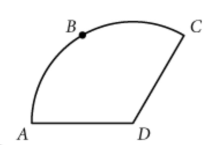# SAT官方每日一题附答案和解析[数学](2020年3月4日)评论打印收藏本文

Mathematics>Standard Multiple ChoiceThe figure on the left shows a sector of a circle with center D. If the area of the sector is 500 square units and the length of arc ABC is 40 units, what is the radius of the circle?
The formula for the area of a circular sector is A=1/2θr^2 , where A is the area, θ is the central angle of the sector in radians, and r is the radius.

The formula for the length of a circular arc is s=rθ, where s is the arc length, r is the radius, and θ is the central angle formed by the arc in radians.

A.12.5
B.16
C.20
D.25

 重点单词 查看全部解释 figure ['figə] 想一想再看 n. 图形，数字，形状; 人物，外形，体型v 联想记忆 radius ['reidiəs] 想一想再看 n. 半径，桡骨，半径范围，辐射区 联想记忆 circle ['sə:kl] 想一想再看 n. 圈子，圆周，循环v. 环绕，盘旋，包围 multiple ['mʌltipl] 想一想再看 adj. 许多，多种多样的n. 倍数，并联 联想记忆 circular ['sə:kjulə] 想一想再看 adj. 循环的，圆形的n. 传单，通报 联想记忆 formula ['fɔ:mjulə] 想一想再看 n. 公式，配方，规则；代乳品adj. （赛 联想记忆
﻿# 可可英语官方微信(微信号:ikekenet)

每天向大家推送短小精悍的英语学习资料.添加方式1.扫描上方可可官方微信二维码。
添加方式2.搜索微信号ikekenet添加即可。